# gkrecall

We brings you daily Current affairs, daily Current Affairs Quiz, weekly Current Affairs, weekly Current Affairs Quiz to enhance your preparation for upcoming exams. We also provide articles related to quant, reasoning, English, ssc etc .

## Breaking

We bring you the complete and important daily  Quant Quiz to achieve more marks in Banking, Insurance, UPSC, SSC, CLAT, Railways and all other competitive Exams. We prepare it based on our daily current affairs.Hope you like it.

START QUIZ

CASLET

Directions: Ankit owns a shop where he sells 4 different types of products P, Q, R and S. The cost price of product Q is Rs. 2000 which is marked up by 20%. The marked price of product P is Rs. 150 less than the marked price of product Q. The average of the cost price of all 4 products is Rs. 2050. He earns a profit of Rs. 450 on selling product S. The marked price of product S is Rs. 1400 more than the marked price of product R. Ankit offers the discount of 20%, 10% and 15% on products P, Q and R respectively. He also earns the profit of 20%, 30% and 15% on products P, R and S respectively.

1.Find the discount percentage offered by Ankit on product D ?

13%

13.16%

11.25%

14.50%

15.40%

2.Had he sold product R at a loss of 10%, then what would have been the selling price of product R ?

1900

1850

1790

1600

1530

3.Find the total profit earned by Ankit on selling all the 4 products ?

1100

1500

1700

1760

1420

4.Find the difference between the selling price of product R and product S ?

1240

1260

1270

1290

1340

5.Let the selling price of product T is (100 / 9) % more than the selling price of product Q. If it is sold after giving two successive discounts of 10% and 16.67%, then find the marked price of product T ?

4000

4500

3000

3200

2800

DATA INTERPRETATION

6.If ratio of literate and illiterate males in city B is 4:3 respectively, find the percentage of literate females in city B.

a) 50.22%

b) 75.12%

c) 33.33%

d) 65.13%

e) None of these

7) Out of the illiterate population of city D, 30% are females. Find the percentage of literate males in city D.

a) 60%

b) 55%

c) 70%

d) 45%

e) None of these

8) Find the respective ratio of number of males in city A number of illiterates in city C.

a) 123: 128

b) 125: 128

c) 123: 125

d) 125: 127

e) None of these

9) Find the total number of literates in all the cities together.

a) 20400

b) 21600

c) 22500

d) 23700

e) None of these

10) Find the difference between total number of males and total number of females in all the cities.

a) 5300

b) 4200

c) 6200

d) 4800

e) None of these

Yacht P is going ___(1) __ at a speed of ___(2) __ km/hr from point M in a stream of speed ___(3) __ km/hr thus the speed of yacht in still water is ___(4) __ km/hr. After travelling a distance of ___(5) __ in ___(6) __ hours it reaches point N it turns around and reaches the starting point in ___(7) __ hours. When the Yacht P leaves point M, Yacht Q at point N starts travelling ___(8) __.

11. If (1) is upstream and (2) and (3) is 18 and 4 respectively, then what is speed downstream?

30 km/hr

14 km/hr

26 km/hr

10 km/hr

12.If (1) is upstream and (3) is 3 and (4) is 30, what is (5) if (6) is 12?

324 km

360 km

396 km

432 km

13.If (1) is downstream, (4) is 24 and (3) is 4. With (5) being 224 km and while coming back (4) decreased by 4 km/hr, what is the value of (6) + (7)?

15 1/3 hr

22 hr

32 hr

10 hr

14.(1) is upstream (4) and (3) is 28 and 4 respectively. (5) is 630 km and speed of Yacht Q in still water is less than (4) by 2 with (8) being at same time. After how many hours do both the Yachts meet?

9 2/3 hr

5 6/13 hr

12 1/3 hr

11 2/3 hr

15.(1) is downstream (4) and (3) is 35 and 3 respectively. (5) is 868 km and the speed of Yacht Q in still water is more than (4) by 2 with (8) after two hours. After how many hours do both the Yacht meet?

18 hours

5 hours

13 hours

11 1/7 hours

QUANTITY COMPARISION

In the following question, two statements are numbered as A and B. On solving these statements we get quantities A and B respectively. Solve for the quantities and choose the correct option.

Quantity A > Quantity B

Quantity A = Quantity B

Quantity A < Quantity B

Quantity A >=  Quantity B

Quantity A  <= Quantity B

16.

Quantity A: A shopkeeper earns a profit of 32%, if he sells a product for Rs. 198. What will be his profit percentage, if he sells the product for Rs. 180 ?

Quantity B: A shopkeeper marks the price of a product 60% above its cost price and sells it at a discount of 20%. What is his profit percentage ?

17.

Quantity A: The simple interest on a certain sum is what percentage of the sum at a rate of 6% p.a. after 10 years ?

Quantity B: The interest compounded annually on a certain sum is what percentage of the sum at a rate of 10% p.a. after 2 years ?

18.

I. a² – 0.89a + 0.133 = 0

II. b² – 2.2b + 1.05 = 0

19.

Quantity A: Area of a rectangle (in cm²) having sides in ratio 4 : 5 and difference of sides 10 cm.

Quantity B: Area of a circle (in cm) having circumference 66 cm\

20.

Find the present age of Tipendra.

Quantity A: Three years before, the ratio of ages of Tipendra and Champaklal was 5 : 6. Three years hence this ratio will become 6 : 7.

Quantity B: 10 years before the ratio of ages of Tipendra and Champaklal was 1 : 3 and 10 years hence the ratio will become 1 : 2.

ARITHMETIC SUM

21.Ten years ago, the age of Bhoomi was three times the age of Shailendra. After ten years, Bhoomi's  age will be twice the age of Shailendra. Present age of Bhoomiis_____years. Shailendra’s age after 15 years is ______

72, 40

74, 25

56, 20

70, 45

22.The common difference of arithmetic progression is _______ if the difference between the 74th term and 36th term is 418 and the first term of the progression is 25. The average of the first 70 terms of the arithmetic progression is _______.

Which of the following options satisfies the two blanks in the question?

A. 11, 404.5

B. 25, 414.5

C. 13, 408.5

D. 15, 407.5

23.In a box, 4 rubber bands of ten rupees, 2 rubber bands of five rupees, 2 rubber bands of two rupees and 2 rubber bands of one rupee are put. Now, three rubber bands are taken out randomly. What is the probability that the rubber bands drawn are of 12 rupees?

1/20

1/10

3/16

1/6

24.Meera, Pyari and Walt invested Rs. ___, Rs. ___ and Rs. 40000 in a business respectively. After 6 months, they again invested Rs. 20000, Rs. 25000 and Rs. 20000 respectively. At the end of the year, they received Rs. 3600, Rs. 4050 and Rs. 3000 respectively as their profit shares.

Which of the following options satisfies the two blanks in the question?

A. 25000, 18000

B. 22000, 33000

C. 45000, 50000

D. 50000, 55000

25.A machine is made up of 4 components Bearings, Axles, Splines and Fasteners. The cost of these components are in the ratio 3 : 4 : 5 : 6 respectively. After 1 year, there has been a percentage change in the costs of these components i.e., 10% increase of Bearings, 20% decrease of Axles, 30% decrease of Splines and 40% increase of Fasteners. Find the percentage change in the cost of the equipment.

1.67% decrease

2.23% increase

3.34% increase

1.67% increase

SOLUTIONS :

If we solve the case step by step, we get the following output.

1.⇒ Discount % offered on product S = (Discount / MP) × 100

⇒ Discount % =450/4000 × 100

⇒ 11.25%

So ,the correct answer is 11.25%

2. Cost price of product R = Rs. 1700

⇒ If it is sold at a loss of 10%

⇒ Selling price = 90/100 × 1700

⇒ Selling price = Rs. 1530

So, the correct answer is Rs. 1530.

3.⇒ Total profit earned = 300 + 160 + 510 + 450

⇒ Rs. 1420

So ,the correct answer is Rs. 1420.

4.⇒ Selling price of product R = Rs. 2210

⇒ Selling price of product S = Rs. 3450

⇒ Difference = 3450 – 2210

⇒ Rs. 1240

So ,the correct answer is Rs. 1240.

5.Selling price of product Q = Rs. 2160

Selling price of product T =

⇒ 2160 + 240

Week 3: Apr 2 - 8

∵ It has been given two successive discounts of 10% and 16.67%

⇒0.9*0.833*Marked price = 2400

⇒ Marked price of product T = 2400 ÷ 0.75

⇒ Marked price of product T = Rs. 3200

So ,the correct answer is Rs. 3200

Number of males in city B = 7/13 x 5200 = 2800

Number of females in city B = 6/13 x 5200 = 2400

Total number of literates in city B = 85/100 x 5200 = 4420

Literate males in city B = 4/7 x 2800 = 1600

Literate females in city B = 2400 – 1600 = 800

Percentage of literate females = 800/2400 x 100 = 33.33%

Illiterate population of city D = (100 – 75)/100 x 4800

= 25/100 x 4800

= 1200

Number of illiterate females = 30/100 x 1200 = 360

Number of illiterate males = 1200 – 360 = 840

Number of males in city D = 7/12 x 4800 = 2800

Number of literate males = 2800 – 840 = 1960

Percentage of literate males = 1960/2800 x 100

= 70%

Number of males in city A = 5/9 x 4500 = 2500

Number of illiterates in city C = (100 – 60)/100 x 6400

= 40/100 x 6400

= 2560

Required ratio = 2500: 2560 = 125: 128

Number of literates in city A = 56/100 x 4500 = 2520

Number of literates in city B = 85/100 x 5200 = 4420

Number of literates in city C = 60/100 x 6400 = 3840

Number of literates in city D = 75/100 x 4800 = 3600

Number of literates in city E = 70/100 x 5600 = 3920

Number of literates in city F = 90/100 x 6000 = 5400

Required sum = 2520 + 4420 + 3840 + 3600 + 3920 + 5400 = 23700

Total number of males in all the cities = 5/9 x 4500 + 7/13 x 5200 + 5/8 x 6400 + 7/12 x 4800 + 4/7 x 5600 + 3/5 x 6000

= 2500 + 2800 + 4000 + 2800 + 3200 + 3600

= 18900

Total number of females in all the cities = 4/9 x 4500 + 6/13 x 5200 + 3/8 x 6400 + 5/12 x 4800 + 3/7 x 5600 + 2/5 x 6000

= 2000 + 2400 + 2400 + 2000 + 2400 + 2400

= 13600

Required difference = 18900 – 13600 = 5300

11.Yacht P is going upstream

Speed Upstream = 18 km/hr

Speed of stream = 4 km/hr

Speed Upstream = Speed of Yacht in still water - Speed of stream

18 = Speed of Yacht in still water - 4

Speed of Yacht in still water = 22 km/hr

Speed downstream = Speed of Yacht in still water + Speed of stream = 22 + 4 = 26 km/hr

12.Yacht P is going upstream

Speed of stream = 3 km/hr

Speed of Yacht in still water = 30 km/hr

Total time taken = 12 hours

Speed upstream = Speed of Yacht in still water - Speed of stream = 30 - 3 = 27 km/hr

Distance travelled = (Speed upstream) (Total time taken)

Distance travelled = 27 × 12 = 324 km

13.Initially, Yacht P is going downstream

Speed of Yacht in still water = 24 km/hr

Speed of stream = 4 km/hr

Distance between P and Q = 224 km

Speed downstream = Speed of Yacht in still water + Speed of stream = 24 + 4 = 28 km/hr

Time taken to go from M to N =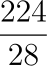= 8 hours

While coming back

Yacht P is going upstream

Speed of Yacht in still water = 24 - 4 = 20 km/hr

Speed of stream = 4 km/hr

Speed upstream = Speed of Yacht in still water - Speed of stream = 20 - 4 = 16 km/hr

Time taken to go from N to M =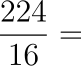14 hours

Thus, total time taken by Yacht P = 8 + 14 = 22 hours

14.Yacht P goes upstream

Speed of Yacht P in still water = 28 km/hr

Speed of stream = 4 km/hr

Speed upstream = 28 - 4 = 24 km/hr

Distance between P and Q = 630km

Yacht Q goes downstream

Speed of Yacht Q in still water = 28 - 2 = 26 km/hr

Speed downstream = 26 + 4 = 30 km/hr

Both the Yachts leave at same time

When the Yacht will meet, Yacht P will travel a distance say x and Yacht Q will travel a distance 630 - z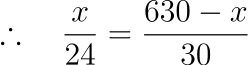∴ x = 280km

Time at which they meet =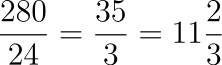hours

15.Yacht P is going downstream

Speed of Yacht P in still water = 35 km/hr

Speed of stream = 3 km/hr

Speed downstream = 35 + 3 = 38 km/hr

Distance between P and Q = 868 km

Yacht Q goes upstream

Speed of Yacht Q in still water = 35 + 2 = 37 km/hr

Speed upstream = 37 - 3 = 34 km/hr

Yacht Q leaves 2 hours later

Thus when the two Yacht meet

Yacht P has travelled x km in t hours

Yacht Q has travelled 868 - x km in t - 2 hours

Thus, distance travelled by Yacht P + distance travelled by Yacht Q = Total distance between point M and N

∴ (Speed downstream of Yacht P) (Time taken by Yacht P) + (Speed upstream of Yacht Q) (Time taken by Yacht Q) = x + 868 - x

∴ (38)(t) + (34)(t - 2) = 868

∴ 38t + 34t - 68 = 868

∴ 72t = 936

∴ t = 13 hours

16.According to question

Considering the first equation,

For Quantity A :

Let the cost price of the product be Rs. ‘x’

∵ Cost price + Profit = Selling price

⇒ x + 32% of x = 198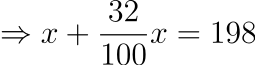⇒ 1.32x = 198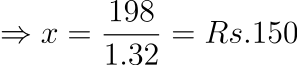So, the cost price of the product is Rs. 150

Now, In the second case

When the selling price = Rs. 180,

⇒ Profit earned = selling price - cost price

⇒ Profit earned = 180 – 150 = Rs. 30

⇒ Profit percentage =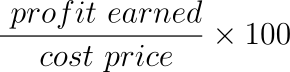⇒ Profit percentage = 30/150 × 100 = 20%

⇒ Quantity A = 20%

Now, consider the second quantity

Let the cost price of the product be Rs. ‘x’

⇒ Marked price = x + 60% of x = 1.6x

⇒ Discount = marked price - 20% of marked price

⇒ Discount = 1.6x – 20% of 1.6x = 1.6x – 0.32x = 1.28x

⇒ Selling price = Marked price – Discount

⇒ Selling price = 1.6x – 0.32x = 1.28x

⇒ Profit earned = selling price - cost price

⇒  Profit earned = 1.28x – x = 0.28x

⇒ Profit percentage = 0.28x/x × 100 = 28%

⇒ Quantity B = 28%

So ,the correct answer is Quantity A < Quantity B.

17.According to question

Considering the first equation,

For Quantity A :

Given : rate (r) = 6% ,time(t) = 10 years

So ,the simple interest is given as

Simple Interest =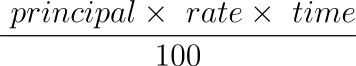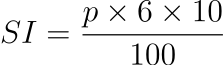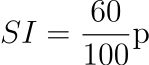So ,SI is 60% of p       ……….eqn(1)

Now ,considering the second quantity

For Quantity B :

Given : rate (r) = 10% ,time (t) = 2 years

So ,the compound Interest is given as

Compound Interest =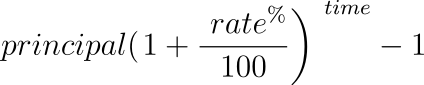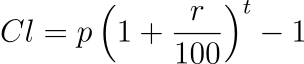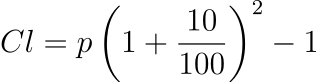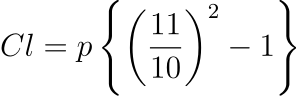CI = 0.21p

So ,CI is 21% of p    ……...eqn (2)

Now from equation (1) & (2) ,we get

Quantity A > Quantity B

So ,the correct answer is Quantity A > Quantity B.

18.Consider the first equation :

⇒ a² – 0.89a + 0.133 = 0

⇒ a² – 0.19a – 0.7a + 0.133 = 0

⇒ a(a – 0.19) – 0.7(a – 0.19) = 0

⇒ (a – 0.7)(a – 0.19) = 0

⇒ a = 0.19 and 0.7            ---- eqn(1)

Now ,consider the second equation

⇒ b² – 2.2b + 1.05 = 0

⇒ b(b – 1.5) – 0.7(b – 1.5) = 0

⇒ (b – 0.7)(b – 1.5) = 0

⇒ b = 0.7 and 1.5               ----eqn(2)

Now ,From eqn (1) and (2)

∴ a ≤ b

19. First we will find Quantity A,

Quantity A:

Since the sides of the rectangle are in the ratio 4 : 5, let the sides be 4x and 5x.

given, 5x - 4x = 10

⇒ x = 10

⇒ sides of the rectangle are 5x = 50 and 4x = 40 respectively.

We know that, area of a rectangle = length × breadth

∴ Area = 50 × 40

⇒ Area = 2000 cm²

Quantity A = 2000

Now,

Quantity B:

Circumference of a circle of radius r = 2πr

⇒ 66 = 2 × (22/7) × r

⇒ r = 10.5 cm

Area of a circle of radius r =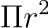∴ Area = (22/7) × (10.5)²

⇒ Area = 346.5²

Quantity B = 346.5

Clearly, Quantity B < Quantity A

20. Quantity A:

Three years before, the ratio of ages of Tipendra and Champaklal was 5 : 6,

Three years hence this ratio will become 6 : 7,

⇒ 5 : 6

⇒ 6 : 7

⇒ Difference in ratio for Tipendra = 1 and Difference in years = 6

⇒ 1 = 6

⇒ Present age of Tipendra = 5 × 6 + 3 = 33 years

Quantity B:

10 years before the ratio of ages of Tipendra and Champaklal was 1 : 3,

10 years hence the ratio will become 1 : 2 = 2 : 4,

⇒ 1 : 3

⇒ 2 : 4

⇒ Difference in ratio for Tipendra = 1 and Difference in years = 20

⇒ 1 = 20

⇒ Present age of Tipendra = 1 × 20 + 10 = 30 years

∴ Quantity A > Quantity B

21.10 years ago, the age of Bhoomi was three times the age of Shailendra. Say, the age of Shailendra was x and Bhoomi's  age was 3x.

At present: Bhoomi's  age is (3x + 10) and Shailendra’s age is (x + 10)

After ten years: Bhoomi's  age will be (3x + 10) +10 and

Shailendra’s age will be (x + 10) + 10.

Given that, Bhoomi's  age is twice that of Shailendra after ten years.

⇒(3x + 10) +10 = 2 [(x + 10) + 10]

⇒ (3x + 20) = 2[x + 20]

Solving the equation, we get x = 20

Bhoomi's  present age = 3 × 20 + 10 = 70 years.

Shailendra’s age after 15 years = 20 + 10 + 15 = 45 years

22.Let a = first term and d = common difference

⇒ Nth term = a + (N – 1)d

Given,

⇒ 418 = a + (74 – 1)d – a – (36 – 1)d

⇒ 418 = 73d – 35d

⇒ d = 11

Common difference is 11.

⇒ a = 25

⇒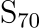= (70/2) × [2 × 25 + (70 – 1)11]

⇒= (35) × [50 + 69 × 11]

⇒= (35) × 

⇒= 28315

∴ Required average = 28315/70

= 404.5

23.

There are 4 rubber bands of ten rupees, 2 rubber bands of five rupees, 2 rubber bands of two rupees and 2 rubber bands of one rupee.

Total rubber bands = 4 + 2 + 2 + 2 = 10

Total number of ways in which 3 rubber bands can be taken out =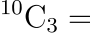= 120

No. of rubber bands drawn can be of 12 rupees in the following cases:

i) 1 rubber band of ten rupees and 2 rubber bands of 1 rupee

This can be done in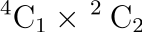ways, i.e. 4 x 1 = 4 ways.

ii) 2 rubber bands of five rupees and 1 rubber band of 2 rupees

This can be done in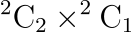ways, i.e. 1 x 2 = 2 ways.

Total number of ways in which 12 rupees of rubber bands can be drawn = 4 + 2 = 6.

∴ Probability of drawing 12 rupees of rubber bands = (Total number of ways in which 10 rupees of rubber bands can be drawn/ Total number of ways in which 3 rubber bands can be drawn) = 6/120 = 1/20.

24.Let Meera and Pyari invested Rs. ‘x’ and Rs. ‘y’ respectively

∵ Meera invested Rs. 20000 after 6 months

⇒ Meera’s total capital investment = 6x + 6 × (x + 20000) = 12x + 120000

∵ Pyari invested Rs. 25000 after 6 months

⇒ Pyari’s total capital investment = 6y + 6 × (y + 25000) = 12y + 150000

∵ Walt invested Rs. 20000 after 6 months

⇒ Walt’s total capital investment = 6 × 40000 + 6 × 60000 = 240000 + 360000 = 600000

∵ Ratio of capital investments = Ratio of profit shares

Considering ratios of Meera and Walt,

⇒ (12x + 120000)/600000 = 3600/3000

⇒ (x + 10000)/50000 = 6/5

⇒ 5x + 50000 = 300000

⇒ x = 250000/5 = Rs. 50000

Considering ratios of Pyari and Walt,

⇒ (12y + 150000)/600000 = 4050/3000

⇒ 12y + 150000 = 810000

⇒ y = 660000/12 = Rs. 55000

∴ Option D satisfies the given blanks

25.Since the cost of these components are in the ratio 3 : 4 : 5 : 6 respectively

Suppose the costs of components Bearings, Axles, Splines and Fasteners are 300, 400, 500 and 600

⇒ The cost of the equipment = 300 + 400 + 500 + 600 = 1800

After one year

⇒ New cost of Bearings = 300 × 1.1 = 330

⇒ New cost of Axles = 400 × 0.8 = 320

⇒ New cost of Splines = 500 × 0.7 = 350

⇒ New cost of Fasteners = 600 × 1.4 = 840

⇒ The cost of the equipment = 330 + 320 + 350 + 840 = 1840

∴ Percentage increase in the cost = [1840 – 1800] / 1800 = 2.23%